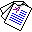# Inductive Equivalence in Clausal Logic and Nonmonotonic Logic Programming

## Chiaki Sakama and Katsumi Inoue

Machine Learning, 83:1-29, Springer-Verlag, 2011.

## Abstract

This paper provides a logical framework for comparing inductive capabilities among agents having different background theories. A background theory is called inductively equivalent to another background theory if the two theories induce the same hypotheses for any observation. Conditions of inductive equivalence change depending on the logic of representation languages and the logic of induction or inductive logic programming (ILP). In this paper, we consider clausal logic and nonmonotonic logic programs as representation languages for background theories. Then we investigate conditions of inductive equivalence in four different frameworks of induction, cautious induction, brave induction, learning from satisfiability, and descriptive induction. We observe that several induction algorithms in Horn ILP systems require weaker conditions of equivalence under restricted problem settings. We address that inductive equivalence can be used for verification and evaluation of induction algorithms, and argue problems for optimizing background theories in ILP.Full Paper (pdf 291K) © Springer-Verlag (The original publication is available at www.springerlink.com)